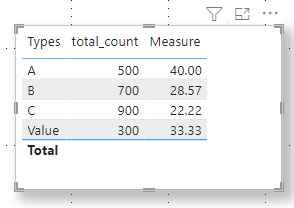cancel
Showing results for
Did you mean:New Member

## How to use SELECTEDVALUE into DAX?

Hello,

I would like to return a value into a column depending on the selected value into a visual slicer, something like this:

Return
(IF(SELECTEDVALUE('<table>'[Types])=="Value", DIVIDE(100*var1_value, <table>[total_value]), Divide ( 100 * var2_count , <table>[total_count] )))

But it seems SELECTEDVALUES doesn't return any value, so the column always returns the false IF result.

Where am I wrong?
1 ACCEPTED SOLUTIONCommunity Support

Hi @Log2Ins

SELECTEDVALUE() : Returns the value when the context for columnName has been filtered down to one distinct value only. Otherwise returns alternateResult.

I created a measure with your formula .

``````Measure =
VAR _value1=100
var _value2=200
Return (IF(SELECTEDVALUE('Table'[Types])="Value", DIVIDE(100*_value1,SELECTEDVALUE('Table'[total_count])), Divide ( 100 * _value2 , SELECTEDVALUE('Table'[total_count]))))``````

You can refer to my measure to change your formula .

The final result is as shown :Best Regards,
Community Support Team _ Ailsa Tao
If this post helps, then please consider Accept it as the solution to help the other members find it more quickly.

2 REPLIES 2Community Support

Hi @Log2Ins

SELECTEDVALUE() : Returns the value when the context for columnName has been filtered down to one distinct value only. Otherwise returns alternateResult.

I created a measure with your formula .

``````Measure =
VAR _value1=100
var _value2=200
Return (IF(SELECTEDVALUE('Table'[Types])="Value", DIVIDE(100*_value1,SELECTEDVALUE('Table'[total_count])), Divide ( 100 * _value2 , SELECTEDVALUE('Table'[total_count]))))``````

You can refer to my measure to change your formula .

The final result is as shown :Best Regards,
Community Support Team _ Ailsa Tao
If this post helps, then please consider Accept it as the solution to help the other members find it more quickly.Super User

@Log2Ins SELECTEDVALUE only returns a value if you have one selection. Otherwise it returns it's alternative result which by default is BLANK. Also, I think you only want one =

``Return (IF(SELECTEDVALUE('<table>'[Types])="Value", DIVIDE(100*var1_value, <table>[total_value]), Divide ( 100 * var2_count , <table>[total_count] )))``

Become an expert!: Enterprise DNA
External Tools: MSHGQM
Latest book!:
Mastering Power BI 2nd EditionDAX is easy, CALCULATE makes DAX hard...